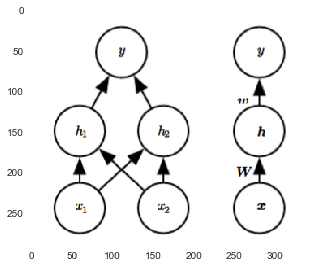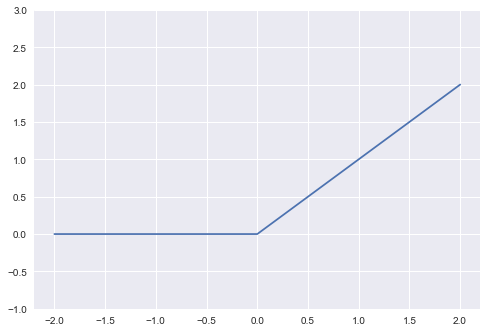In :
# %load /Users/facaiyan/Study/book_notes/preconfig.py
%matplotlib inline

import matplotlib.pyplot as plt
import seaborn as sns
sns.set(color_codes=True)
plt.rcParams['axes.grid'] = False

import numpy as np

#from IPython.display import SVG
def show_image(filename, figsize=None, res_dir=True):
if figsize:
plt.figure(figsize=figsize)

if res_dir:
filename = './res/{}'.format(filename)



# Chapter 6 Deep Feedforward Networks¶

feedforward: no feedback connections.

input layer -> hidden layers -> output layer

\begin{align} y &= f(x; \theta) \approx f^*(x) \\ &= W^T \phi(x) + b \end{align}

how to choose the mapping $\phi$?

• use a very generic $\phi$, such as RBF kernel $\implies$ generation remains poor.
• manually engineer $\phi$ $\implies$ classical feature engineer.
• learn $\phi$ by deep learning. $y = f(x; \theta, w) = \phi(x; \theta)^T w$.
• advantage: only needs to find the right general function family VS find the right function.

### 6.1 Example: Learning XOR¶

$h = g(W^T x + c)$, where an affine transformation followed by an activation function $g$.

In :
show_image("fig6_2.png", figsize=(5, 8))default activation function is rectified linear unit (ReLU): $g(z) = max\{0, z\}$

1. piecewise linear function: very close to linear.
2. easy to optimize with gradient-base methods.
In :
relu = lambda x: np.maximum(0, x)

x = np.linspace(-2, 2, 1000)
y =  relu(x)

plt.ylim([-1, 3])
plt.grid(True)
plt.plot(x, y)

Out:
[<matplotlib.lines.Line2D at 0x10464f438>]important:

• initialize all weights to small random values.
• initialize biases to zero or small positive values (push result to right area of ReLU).

#### 6.2.1 Cost Functions¶

In most cases,

• our parametric model defines a distribution $p(y | x; \theta)$,
• simply use the priciple of maximum likelihood.

$\implies$ cross-entropy as the cost function.

##### 6.2.1.1 Learning Conditional Distributions with Maximum Likelihood¶

maximum likelihdood in neural networks => cost function is simply the negative log-likelihood == cross-entropy.

\begin{equation} J(\theta) = - \mathbb{E}_{x, y \sim \hat{p}_{data}} \log p_{model}(y | x) \end{equation}

• Specifying a model p(y | x) automatically determines a cost function log p(y | x). => removes the burden of designing cost functions for each model.
• Undo the exp of some output units => avoid staturate problem (flat area, very small gradient).

unusual property of the cross-entropy cose: does not have a minimum value (negative infinity). => regularization.

##### 6.2.1.2 Learning Conditional Statistics¶

cost L2: learn mean of y when x is given.

cost L1: learn median of y when x is given.

#### 6.2.2 Output Units¶

##### 6.2.2.1 Linear Units for Gaussian Output Distributions¶

Linear output layers are often used to produce the mean of a conditional Gaussian distribution.

##### 6.2.2.2 Sigmoid Units for Bernoulli Output Distributions¶

binary classification

\begin{align} P(y) &= \delta((2y - 1) z) \quad \text{where } z = w^T h + b \\ J(\theta) &= - \log P(y | x) \quad \text{undo exp} \\ &= \zeta ((1 - 2y) z) \end{align}

maximum likelihood is almost always the preferred approach to training sigmoid output units.

##### 6.2.2.3 Softmax Units for Multinoulli Output Distributions¶

multiple classification

\begin{equation} \operatorname{softmax}(z)_i = \frac{\exp(z_i)}{\sum_j \exp(z_j)} \end{equation}\begin{align} \log \operatorname{softmax}(z)_i &= z_i - log \sum_j \exp(z_j) \\ & \approx z_i - \max_j (z_j) \end{align}
• Overall, unregularized maximum likelihood will drive the softmax to predict the fraction of counts of counts of each outcome observed in the training set.

• The argument $z$ can be produced in two different ways:

1. one vs rest: N estimators
2. choose one class as "pivot" class: N - 1 estimators
• softmax provides a "softened" version of the argmax.

##### 6.2.2.4 Other Output Types¶

In general, think neural network as representing a function $f(x; \thetha) = w$, which provides the parameters for a distribution over $y$. Our loss function can then be inperpreted as $- \log p(y; w(x))$.

### 6.3 Hidden Units¶

• Rectified linear units are an excellent default choice of hidden unit.
• In practice, gradient descent still performs well enough for functions which are not actually differentiable.

#### 6.3.2 Logistic Sigmoid and Hyperbolic Tangent¶

The widespread saturation of sigmoidal units => use as hidden units is now discouraged.

### 6.4 Architecture Design¶

• layers: group of units
• chain structure
1. the depth of the network
2. the width of each layer

#### 6.4.1 Universal Approximation Properties and Depth¶

universal approximation theorem: large MLP will be able to represent any function. However, we are not guaranteed that the training algorithm will be able to learn that function.

Empirically, greater depth does seem to result in better generalization.

### 6.5 Back-Propagation and Other Differentiation Algorithms¶

chain rule:

\begin{equation} \frac{dz}{dx} = \frac{dz}{dy} \frac{dy}{dx} \end{equation}

### 6.6 Historical Notes¶

In [ ]: